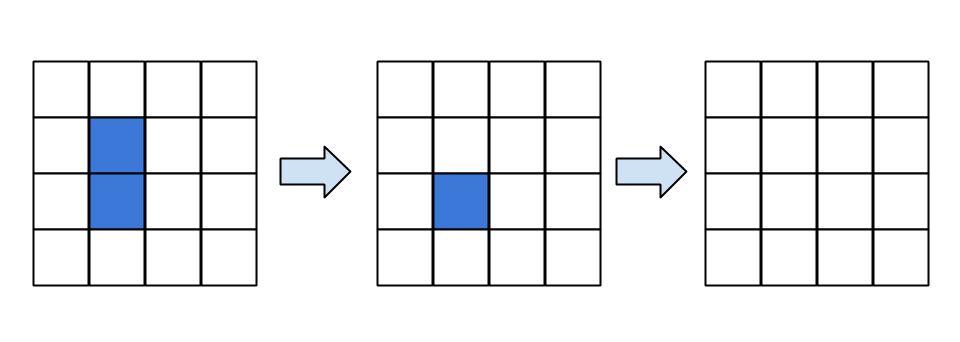# A Paradox of Toom's Rule?

Science is slow.  You can do things like continue a conversation with yourself (and a few commenters) that started in 2005.  Which is what I’m now going to do 🙂  The below is probably a trivial observation for one of the cardinals, but I find it kind of interesting.
Let’s begin by recalling the setup.  Toom’s rule is a cellular automata rule for a two dimensional cellular automata on a square grid.  Put +1 and -1’s on the vertices of a square grid, and then use the following update rule at each step: “Update the value with the majority vote of your own state, the state of your neighbor to the north, and the state of your neighbor to the east.”  A few steps of the rule are shown here with +1 as white and -1 as blue:As you can see Toom’s rule “shrinks” islands of “different” states (taking away such different cells from the north and east sides of such an island.)  It is this property which gives Toom’s rule some cool properties in the presence of noise.
So now consider Toom’s rule, but with noise.  Replace Toom’s update rule with the rule followed by, for each and every cell a noise process.  For example this noise could be to put the cell into state +1 with p percent probability and -1 with q percent probability.  Suppose now you are trying to store information in the cellular automata.  You start out at time zero, say, in the all +1 state.  Then let Toom’s rule with noise run.  If p=q and these values are below a threshold, then if you start in the +1 state you will remain in a state with majority +1 with a probability that goes to one exponentially as a function of the system size.  Similarly if you start in -1.  The cool thing about Toom’s rule is that this works not just for p=q, but also for some values of p not equal to q (See here for a picture of the phase diagram.)  That is there are two stable states in this model, even for biased noise.
Contrast Toom’s rule with a two dimensional Ising model which is in the process of equilibriating to temperature T.  If this model has no external field applied, then like Toom’s rule there is a phase where the mostly +1 and the mostly -1 states are stable and coexist.  These are from zero temperature (no dynamics) to a threshold temperature T, the critical temperature of the Ising model. But, unlike in Toom’s rule, if you now add an external field, which corresponds to a dynamics where there is now a greater probability of flipping the cell values to a particular value (p not equal to q above), then the Ising model no longer has two stable phases.
In fact there is a general argument that if you look at a phase diagram as a function of a bunch of parameters (say temperature and applied magnetic field strength in this case), then the places where two stable regimes can coexist has to be a surface with one less dimension than your parameter space.  This is known as Gibbs’ phase rule.  Toom’s rule violates this.  It’s an example of a nonequilibrium system.
So here is what is puzzling me.  Consider a three dimensional cubic lattice with +1,-1 spins on its vertices. Define an energy function that is a sum over terms that act on the spins on locations (i,j,k), (i+1,j,k), (i,j+1,k), (i,j,k+1) such that E = 0 if the spin at (i,j,k+1) is in the correct state for Toom’s rule applied to spins (i,j,k), (i+1,j,k), and (i,j+1,k) and is J otherwise.  In other words the terms enforce that the ground state locally obey’s Toom’s rule, if we imagine rolling out Toom’s rule into the time dimension (here the z direction). At zero temperature, the ground state of this system will be two-fold degenerate corresponding to the all +1 and all -1 state.  At finite temperature this model well behave as a symmetric noise Toom’s rule model (see here for why.)  So even at finite temperature this will preserve information, like the d>2 Ising model and Toom’s CA rule.
But since this behaves like Toom’s rule, it seems to me that if you add an external field, then this system is in a bit of paradox.  On the one hand, we know from Gibb’s phase rule, that this should not be able to exhibit two stable phases over a range of external fields.  On the other hand, this thing is just Toom’s rule, laid out spatially.  So it would seem that one could apply the arguments about why Toom’s rule is robust at finite field.  But these contradict each other.  So which is it?

This entry was posted in Physics. Bookmark the permalink.